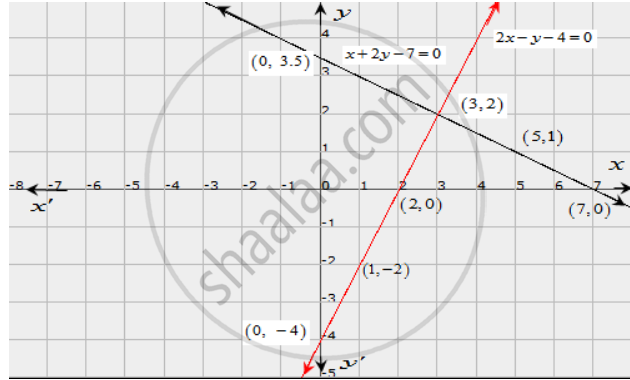Share

# Solve Graphically Each of the Following Systems of Linear Equations. Also, Find the Coordinates of the Points Where the Lines Meet Axis Of Y. X + 2y − 7 = 0, 2x − Y − 4 = 0 - Mathematics

Course

#### Question

Solve graphically each of the following systems of linear equations. Also, find the coordinates of the points where the lines meet axis of y.

x + 2y − 7 = 0,

2x − y − 4 = 0

#### Solution

The given equations are

x + 2y − 7 = 0  ..............(i)

2x − y − 4 = 0  ...............(ii)

The two points satisfying (i) can be listed in a table as,

 x 5 7 y 1 0

The two points satisfying (ii) can be listed in a table as,

 x 2 1 y 0 -2

Now, the graph of equations (i) and (ii) can be drawn as,It is seen that the solution of the given system of equations is given by x = 3, y = 2.

Also, it is observed that the lines (i) and (ii) meet the y-axis at the points (0,3.5) and (0, -4) respectively.

Is there an error in this question or solution?